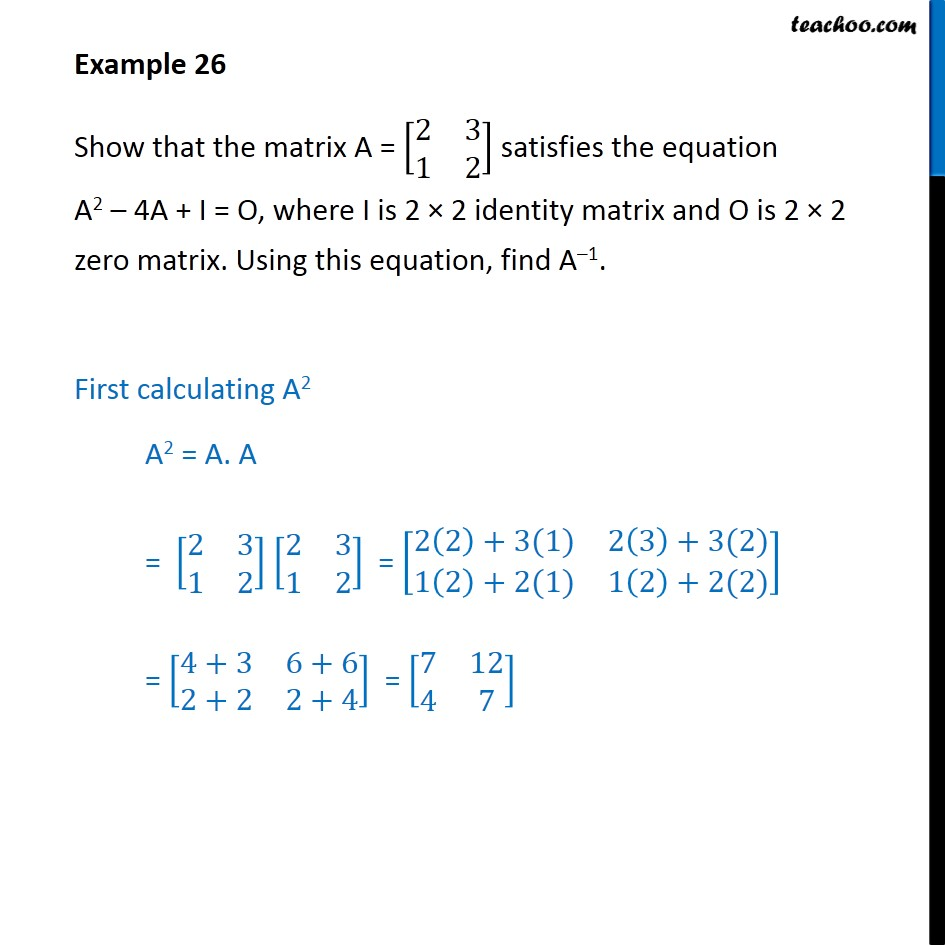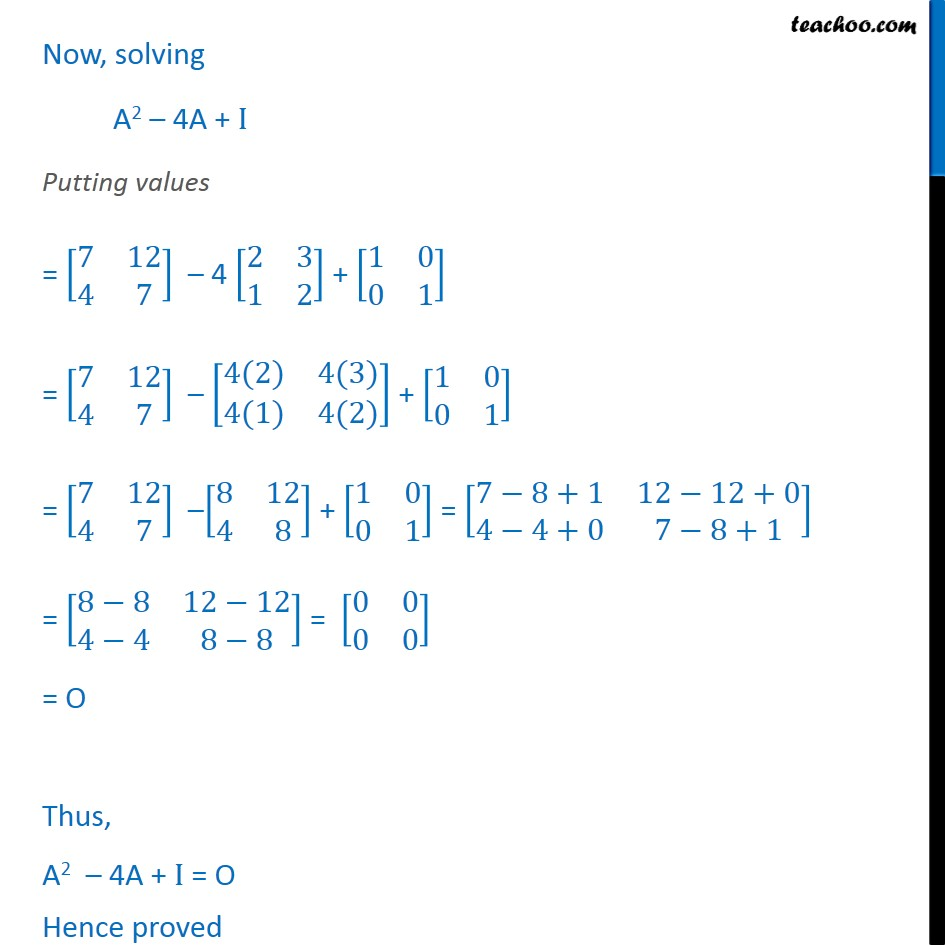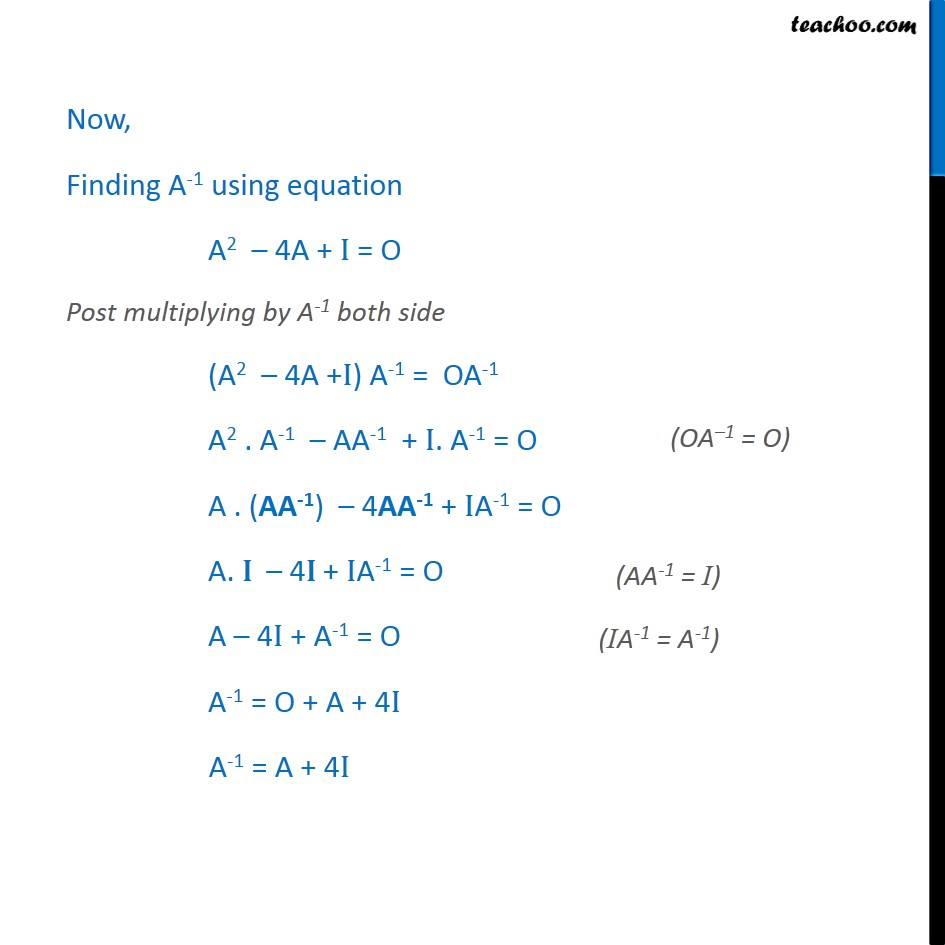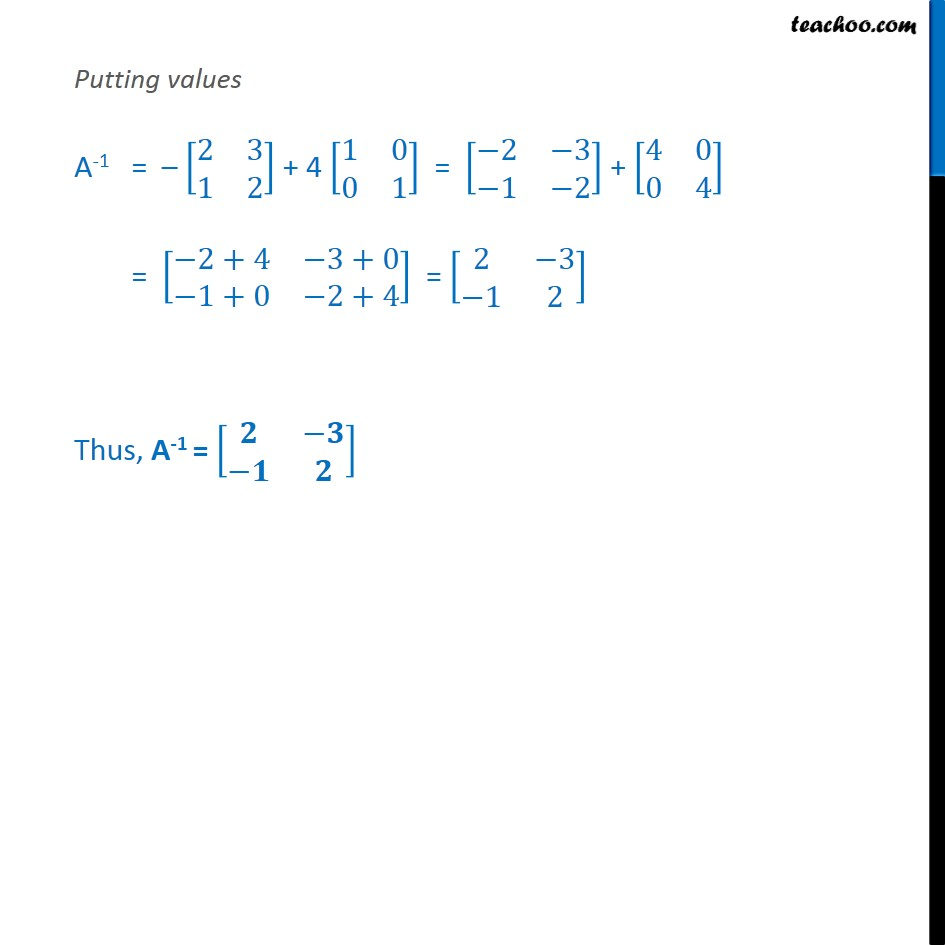1. Class 12
2. Important Question for exams Class 12
3. Chapter 4 Class 12 Determinants

Transcript

Example 26 Show that the matrix A = 2﷮3﷮1﷮2﷯﷯ satisfies the equation A2 – 4A + I = O, where I is 2 × 2 identity matrix and O is 2 × 2 zero matrix. Using this equation, find A–1. First calculating A2 A2 = A. A = 2﷮3﷮1﷮2﷯﷯ 2﷮3﷮1﷮2﷯﷯ = 2 2﷯+3(1)﷮2 3﷯+3(2)﷮1 2﷯+2(1)﷮1 2﷯+2(2)﷯﷯ = 4+3﷮6+6﷮2+2﷮2+4﷯﷯ = 7﷮12﷮4﷮7﷯﷯ Now, solving A2 – 4A + I Putting values = 7﷮12﷮4﷮7﷯﷯ – 4 2﷮3﷮1﷮2﷯﷯ + 1﷮0﷮0﷮1﷯﷯ = 7﷮12﷮4﷮7﷯﷯ – 4(2)﷮4(3)﷮4(1)﷮4(2)﷯﷯ + 1﷮0﷮0﷮1﷯﷯ = 7﷮12﷮4﷮7﷯﷯ – 8﷮12﷮4﷮8﷯﷯ + 1﷮0﷮0﷮1﷯﷯ = 7−8+1﷮12−12+0﷮4−4+0﷮7−8+1﷯﷯ = 8−8﷮12−12﷮4−4﷮8−8﷯﷯ = 0﷮0﷮0﷮0﷯﷯ = O Thus, A2 – 4A + I = O Hence proved Now, Finding A-1 using equation A2 – 4A + I = O Post multiplying by A-1 both side (A2 – 4A +I) A-1 = OA-1 A2 . A-1 – AA-1 + I. A-1 = O A . (AA-1) – 4AA-1 + IA-1 = O A. I – 4I + IA-1 = O A – 4I + A-1 = O A-1 = O + A + 4I A-1 = A + 4I Putting values A-1 = – 2﷮3﷮1﷮2﷯﷯ + 4 1﷮0﷮0﷮1﷯﷯ = −2﷮−3﷮−1﷮−2﷯﷯ + 4﷮0﷮0﷮4﷯﷯ = −2+4﷮−3+0﷮−1+0﷮−2+4﷯﷯ = 2﷮−3﷮−1﷮2﷯﷯ Thus, A-1 = 𝟐﷮−𝟑﷮−𝟏﷮𝟐﷯﷯

Chapter 4 Class 12 Determinants

Class 12
Important Question for exams Class 12

About the AuthorDavneet Singh
Davneet Singh is a graduate from Indian Institute of Technology, Kanpur. He has been teaching from the past 9 years. He provides courses for Maths and Science at Teachoo.